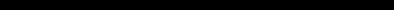# ZX PRINTER OPERATING INSTRUCTIONSThe LPRINT Error A printing error occurs when using LPRINT on decimal numbers in the range .00001 to .0099999999. The leading zeroes, except the first, print as various non-numeric characters instead of zero. This is overcome by converting the number you want to LPRINT into a string. Although the string looks the same, it will print correctly because the routines inside the computer treat strings and numbers differently. Alternatively PRINT on the TV screen then use COPY. Suggested programming alternatives Example 1 Replace: 100 LPRINT A With: 100 LET A\$ = STR\$ A 110 LPRINT A\$ Example 2 Replace: 100 LPRINT A+B/C-D, SIN(1-Q+N) With: 100 LET A\$=STR\$(A+B/C-D) 110 LET B\$=STR\$(SIN(1-Q+N)) 120 LPRINT A\$,B\$ Example 3 Replace: 100 LPRINT "VOLUME="; A*B*C;" LITRES" With: 100 LET A\$=STR\$(A*B*C) 110 LPRINT "VOLUME=";A\$;" LITRES" Note: You only need to change your program if you are likely to LPRINT decimal numbers which would otherwise print incorrectly. This error is restricted to LPRINT, both COPY and LLIST work correctly.